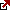# The Doubling Time of Investment Compounded Continuously

## What is doubling time?

Doubling time represents the amount of time for a given quantity to double in size or value at a constant growth rate. Doubling time can be applied in the finance industry, such as inflation, population growth,  resource extraction, consumption of goods, etc.

## The doubling time of investment compounded continuously

How to calculate doubling time?

The doubling time of investment with continuous compounding represents a formula where the natural log of 2 is divided by the rate of return. Using this formula, we can calculate the length of time it takes to double money in an investment account.

Using the doubling time formula with continuous compounding is one of the best ways to calculate the length of time needed to double funds in an investment or account. This formula is used to find the time it takes to double funds. A prime example of this would be monthly rates. If a monthly rate is utilized, the answer will reflect the number of months needed for doubling funds. If an annual rate is used, the answer will reflect the number of years required for doubling funds.

## Doubling time formula

This is a formula where we can find the doubling time of an investment earning :

Doubling Time = ln(2)/r where r is rate

How long to double investment calculator :

You can change the rate in percent. In this example, if annually I have 6% growth, then in 11.5 years, I will double my account. If I have 7% annulary growth, I will double my account in all most 7 years.

## Doubling time formula practical use

There are many practical uses for this formula. One of the primary uses is to discover how long a specific increase in funds will take. The type of increase is a full double. This means that the individual is looking to double the number of funds in their account. The formula will be based upon the amount of time used in the rate. If an individual wants to find how long it will take to double funds with a 6% annual continuous compound, they will take a few steps to find the answer. The first step for solving this equation would be to calculate the number of years needed to double the investment. The annual rate must be used to solve this step in the equation. The answer to this specific equation is 11.5 years. This is one example of how the doubling time formula can be successfully solved with continuous compounding.

It is important to note how the doubling time formula was derived. The first step to understanding this formula is to look at the basic continuous compounding formula. FV represents future value, and PV represents the present value. To successfully double funds, the future value must equal twice the value of the PV. Once this is accomplished, the funds have been doubled. To understand this equation, one can substitute 2 for FV and 1 for the variable of PV. The formula can also be adjusted as well. The formula can also be rewritten to solve for “T.”

It is also important to note that the denominator of the formula also becomes “R.” The doubling time formula has been used for a wide variety of scenarios. It is a common task to solve this equation when looking to double funds. As stated previously, a monthly rate or annual rate may be used to solve this formula fully. There are two main ways to solve this equation. One method involves calculating the number of months, while the other method calculates the number of years. Either method can be used depending upon the specifics. The formula is used with the rate of return. The rate of return plays a role in solving this equation. Investments and accounts are involved with this specific formula and can help solve the equation for doubling time with continuous compounding.

Latest posts by Fxigor (see all)

Fxigor

Igor has been a trader since 2007. Currently, Igor works for several prop trading companies. He is an expert in financial niche, long-term trading, and weekly technical levels. The primary field of Igor's research is the application of machine learning in algorithmic trading. Education: Computer Engineering and Ph.D. in machine learning. Igor regularly publishes trading-related videos on the Fxigor Youtube channel. To contact Igor write on: igor@forex.in.rs

Trade gold and silver. Visit the broker's page and start trading high liquidity spot metals - the most traded instruments in the world.